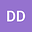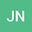Uniqueness for multidimensional kernel determination problems from a parabolic integro-differential equation
••• Durdimurod Durdiev,
• Javlon Nuriddinov
Durdimurod Durdiev
Bukhara State University
Author ProfileJavlon Nuriddinov
Bukhara State University
Author Profile## Abstract

We study two problems of determining the kernel of the integral terms in a parabolic integro-differential equation. In the first problem the kernel depends on time t and x = (x1, …, xn) spatial variables in the multidimensional integro-differential equation of heat conduction. In the second problem the kernel it is determined from one dimensional integro-differential heat equation with a time-variable coefficient of thermal conductivity. In both cases it is supposed that the initial condition for this equation depends on a parameter y = (y1, …, yn) and the additional condition is given with respect to a solution of direct problem on the hyperplanes x = y. It is shown that if the unknown kernel has the form k(x, t) =∑i=oNai(x)bi(t), then it can be uniquely determined.